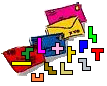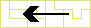Pentomino Quartets and congruent shapes
Helmut Postl from Austria mailed us this nice pentomino problem.
Look for 4 pentominoes A,B,C and D so that A+B and C+D make the same shape.
You also do that with A+C and B+D and then with A+D and B+C.

Example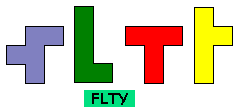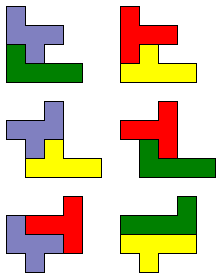Find a solution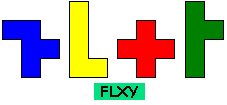Solution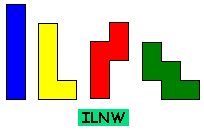Solution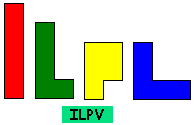SolutionSolution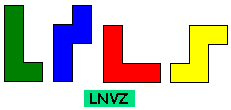Solution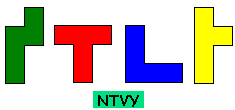Solution

At Christmas 2011 we received an email from George Sicherman: "I just found your page on Pentomino Quartets. For your information, there are 37 quartets in all:
FILP FILY FLPY FLTY FLUY FLWY FLXY FLYZ FNPW FNTY FNVY FNWY FNXY FNYZ FPTY ILNP ILNV ILNW ILPT ILPV ILPY ILTY LNPT LNPU LNPY LNUV LNVZ LPUV LPUY LPVZ LTUY LTYZ NPTW NPTZ NPUY NPWY NTVY
Let me know if you would like to see the solutions. I think you are clever enough to find them on your own!"Solution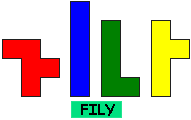Solution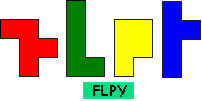Solution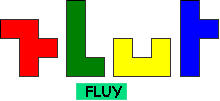Solution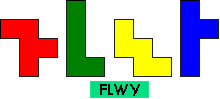Solution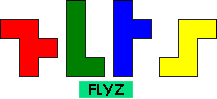SolutionSolution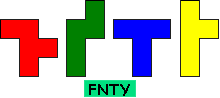Solution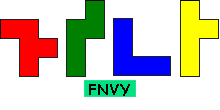SolutionSolution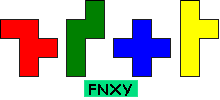Solution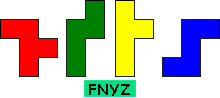Solution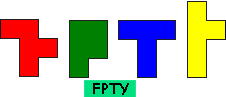SolutionSolution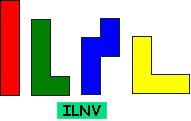Solution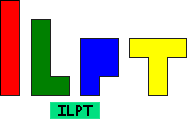SolutionSolution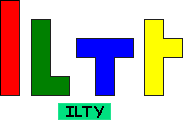Solution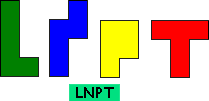SolutionSolutionSolution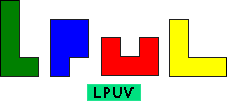Solution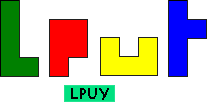Solution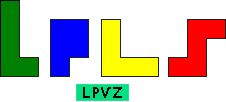SolutionSolution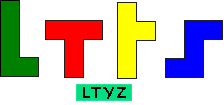Solution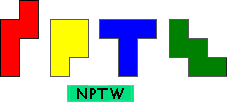Solution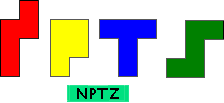Solution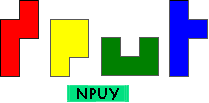Solution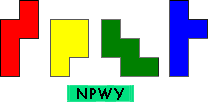Solution

Do you have other groups of 4 pentominos?
Mail us